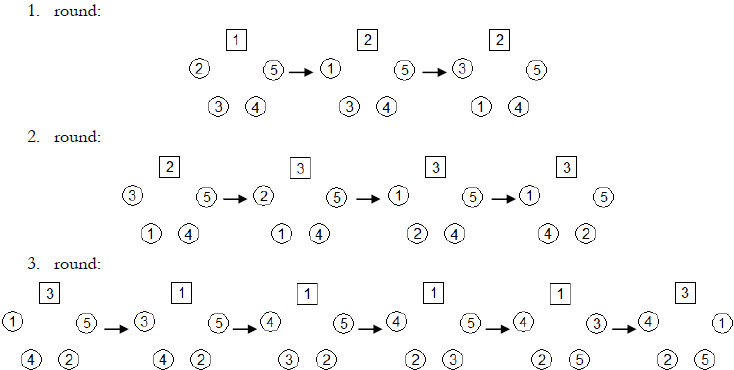### Croation Olympiad in Informatics - 2010

During meetings of young mathematicians a frequent pastime is the Prime Number Circle. For this task, we refer to mathematicians in the circle with numbers 1 to N.

Before the game starts we first draw N-1 circles and one square on the pavement arranged in a big circle. The player numbered 1 stands in the square. All other players stand in the circles, starting with the player 2 in a counterclockwise fashion facing towards the middle of the big circle.

The game consists of K rounds. In the i-th round the person standing in the square jumps up, says "It's me!" and then swaps places with the person standing on his right side pk times, where pk is the k-th prime. For example for N = 5 and K = 3 the following three rounds occur:Write a program that will for given N, K and A determine the neighbours of the player numbered A at the end of the game.

### Input

The first and only line contains three integers N, K and A. (1 ≤ A ≤ N), the number of players, rounds and the selected player.

### Scoring

Test data is divided into four groups each worth 25 points, with the following constraints:

First group: (3 ≤ N ≤ 1 000, 1 ≤ K ≤ 1 000).

Secong group: (3 ≤ N ≤ 1 000, 1 ≤ K ≤ 50 000).

Third group: (3 ≤ N ≤ 50 000, 1 ≤ K ≤ 50 000).

Fourth group: (3 ≤ N ≤ 5 000 000, 1 ≤ K ≤ 500 000).

### Output

The first and only line of output should contain two integers, the numbers on the right and left neighbours of the player numbered A at the end of the game.

`5 3 1`

`3 5`

`5 3 2`

`5 4`

`5 4 5`

### Output

`3 2`

Point Value: 20 (partial)
Time Limit: 1.00s
Memory Limit: 32M